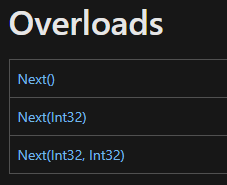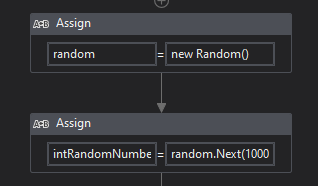# Error is displayed when trying to create 16 digit fixed length random number

Hi all,
Error is displayed when trying to create 16 digit fixed length random number
I am assigning an int variable as:
intRandomNumber= New Random() Next(1000000000000000,9999999999999999)

But its showing error as ‘Constant expression not representable in integer’

Even if i selected variable type as ‘Double’ it’s giving me similar error.

Can anyone kindly help.

Thank you

HiThe next method accepts only Int32 values. Largest int32 number have only 10 digits.

But you can split generating into 2 parts:
generate first 8 digits:

``````random.Next(10000000,99999999)
``````

Convert this number to decimal, multiple by 10000000 and add to second 8-digits random number:

``````random.Next(00000000,99999999)
``````

Now you should have 16-digits random value from 1000000000000000-9999999999999999.
I recommend to use decimal instead of double in this case (double have 15-17 digits precision)

1 Like

To generate a 16-digit random number, you can use a different data type, such as a `Long` or a `Decimal` , which can represent larger values. Here is an example of how you could generate a 16-digit random number using a `Long` data type.

Random random = new Random()
long intRandomNumber = random.Next(1000000000000000L, 9999999999999999L)

Thanks!!!

This alternate method is good. Thank you for bringing it up

Hi @Kaviyarasu_N thanks for the reply.
long datatype is variable type 'Microsoft.Visualbasic.compilerservices.longtype ??

Also how to store this in variable?
Random random = new Random()
long intRandomNumber = random.Next(1000000000000000L, 9999999999999999L)

full name of long type is ‘System.Int64’

You can use assign activitiy:where random variable is of type System.Random

I tried as you told.
But it’s still giving me same error as mentioned in my query
I am using int64 as variable type for the number but still same issue

Hi,

If you can use Windows project (or cross platform project) (this means .Net6), NextInt64 method helps you.

``````longVar = rnd.NextInt64(1000000000000000L,9999999999999999L)
``````

Regards,

Thank you @Yoichi for the reply.
Because of company policy restrictions i cannot use any other cross platform.

But thank you for providing the reason behind

Hi,

In this case, we can achieve it using two Random.Next method as the following.

``````rnd = New Random()
v1 = rnd.Next(0,99999999)
v2 = rnd.Next(10000000,99999999)
``````

then

``````Int64.Parse(v2.ToString+v1.ToString)
``````

Sample20221228-7L.zip (2.2 KB)

Regards,

Yes this alternative solution works @Yoichi .
Thank you

Hi,

Sorry, but need to modify it as the following. It’s better to add PadLeft method as the following, because v1 might has less 8 digits.

``````Int64.Parse(v2.ToString+ v1.ToString.PadLeft(8,"0"c))
``````

Regards,

Hi @Yoichi ,
how do I know, if I have .Net6 or how can I use it (if our company policy allows)?

Regards,
Moritz

HI,

We can check it in project panel or bottom-right corner of Studio.

it depends on Compatibility which we choose in create the project.

Regards,

1 Like

Hi @Yoichi ,